# NCERT Solutions for Class 6 Maths Chapter 8 Decimals

NCERT Solutions for Class 6 Maths Chapter 8 Decimals

### NCERT Solutions for Class 6 Maths Chapter 8 Decimals Ex 8.1

NCERT Solutions for Class 6 Maths Chapter 8 Decimals Ex 8.1

Exercise 8.1

Ex 8.1 Class 6 Maths Question 1.
Write the following as numbers in the given table.Solution:
From the given data, we have

 Hundreds (100) Tens (10) Ones (1) Tenths (1/10) 0 3 1 2 1 1 0 4

Ex 8.1 Class 6 Maths Question 2.
Write the following decimals in the place value table.
(a) 19.4
(b) 0.3
(c) 10.6
(d) 205
Solution:
Place value table is given as under:Ex 8.1 Class 6 Maths Question 3.
Write each of the following as decimals:
(a) Seven-tenths
(b) Two tens and nine-tenths
(c) Fourteen point six
(d) One hundred and two ones
(e) Six hundred point eight
Solution:
(a) Seven-tenths = 7 x 1/10 = 0.7
(b) Two tens and nine-tenths = 2 tens + 0 ones + 9 tenths = 20 + 9/10 = 20.9
(c) Fourteen point six = 14.6
(d) One hundred and two ones
= 1 x 100 + 2 x 1 = 100 + 2 = 102
(e) Six hundred point eight = 600.8

Ex 8.1 Class 6 Maths Question 4.
Write each of the following as decimals: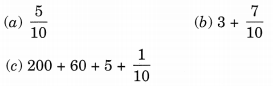Solution:Ex 8.1 Class 6 Maths Question 5.
Write the following decimals as fractions. Reduce the fractions to lowest form.
(a) 0.6
(b) 2.5
(c) 1.0
(d) 3.8
(e) 13.7
(f) 21.2
(g) 6.4
Solution: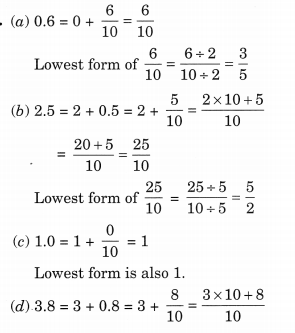Ex 8.1 Class 6 Maths Question 6.
Express the following as cm using decimals.
(a) 2 mm
(b) 30 mm
(c) 116 mm
(d) 4 cm 2 mm
(e) 162 mm
(f) 83 mm
Solution:
We know that 10 mm = 1 cm.Ex 8.1 Class 6 Maths Question 7.
Between which two whole numbers on the number line are the given numbers lie? Which of these whole numbers is nearer the number?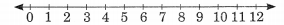(a) 0.8
(b) 5.1
(c) 2.6
(d) 6.4
(e) 9.1
(f) 4.9
Solution:
(a) 0.8 lies between the whole numbers 0 and 1.
(a) 5.1 lies between the whole numbers 5 and 6.
(b) 2.6 lies between the whole numbers 2 and 3.
(c) 6.4 lies between the whole numbers 6 and 7.
(d) 9.1 lies between the whole numbers 9 and 10.
(e) 4.9 lies between the whole numbers 4 and 5.

Ex 8.1 Class 6 Maths Question 8.
Show the following numbers on the number line.
(a) 0.2
(b) 1.9
(c) 1.1
(d) 2.5
Solution:Ex 8.1 Class 6 Maths Question 9.
Write the decimal number represented by the points A, B, C, D on the given number line.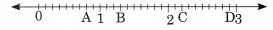Solution:
Point A represents 0.8
Point B represents 1.3
Point C represents 2.2
Point D represents 2.9

Ex 8.1 Class 6 Maths Question 10.
(a) The length of Ramesh’s notebook is 9 cm 5mm. What will be its length in cm?
(6) The length of a young gram plant is 65 mm. Express its length in cm.
Solution:
(a) Length of the notebook = 9 cm 5 mm(b) Length of the young gram plant = 65 mm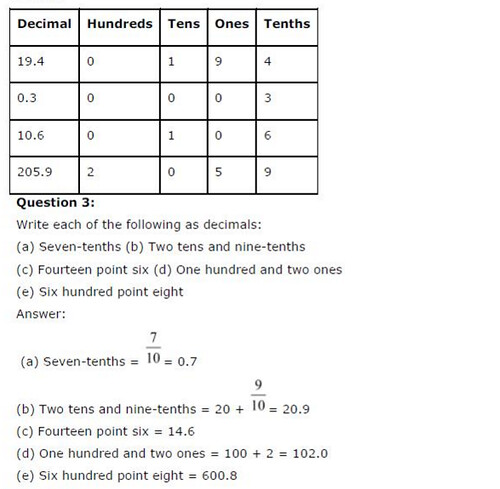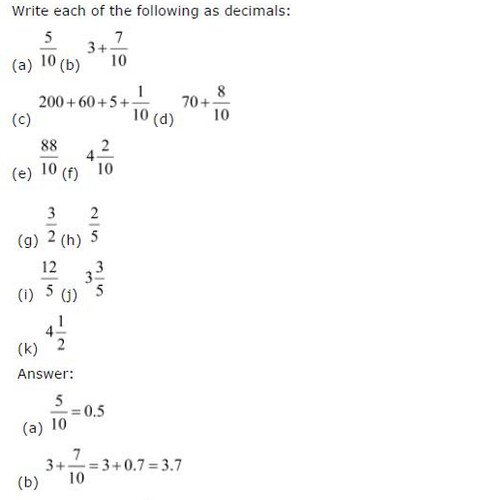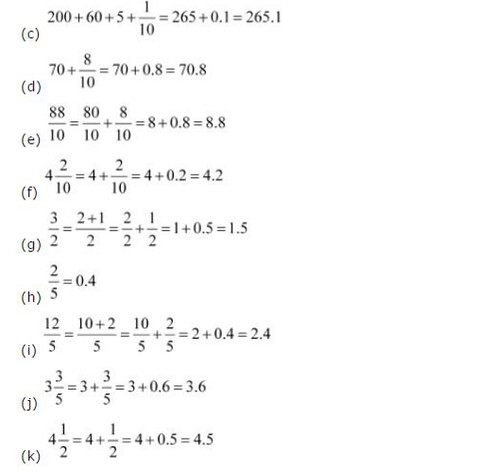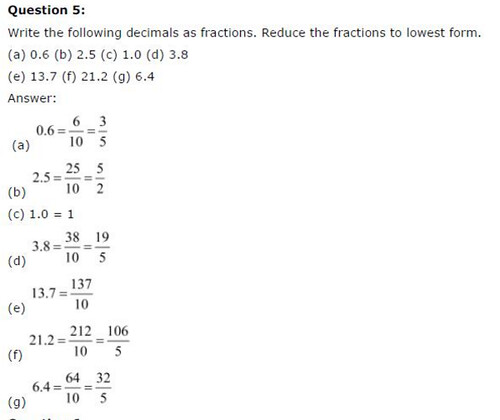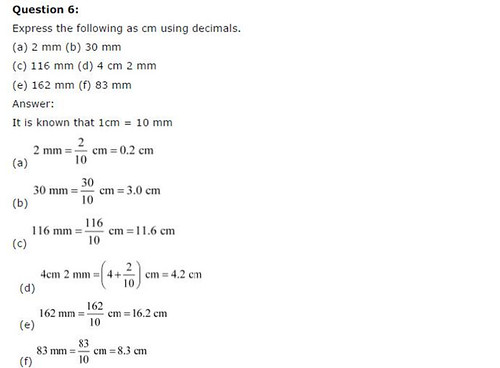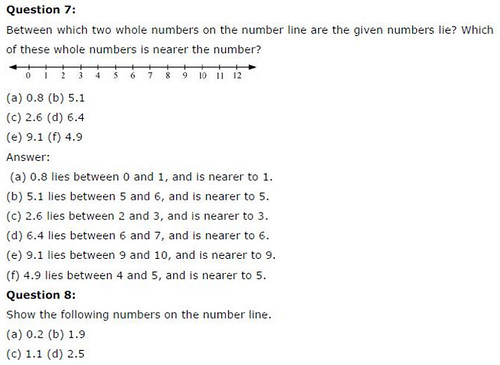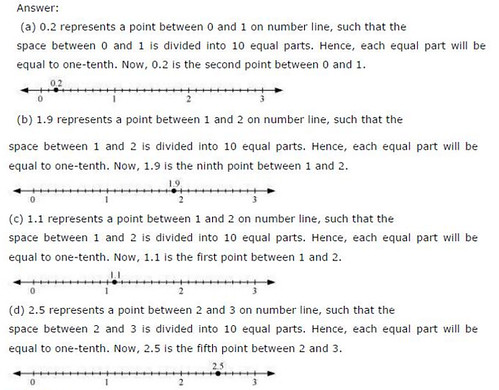## SabDekho

The Complete Educational Website